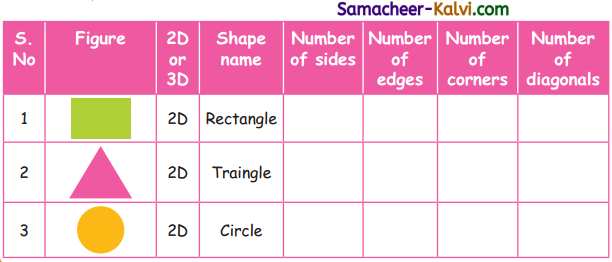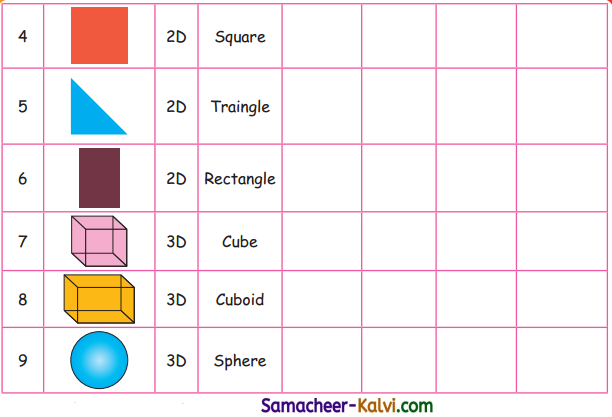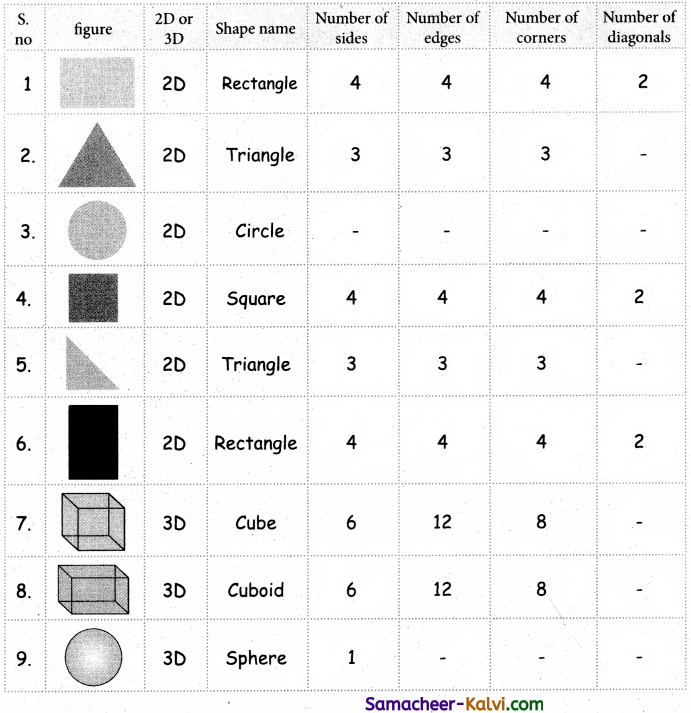## Samacheer Kalvi 3rd Standard Maths Book Solutions Term 1 Chapter 1 Geometry

Practice (Text Book Page No. 4)

Question 1.
Triangle has ______ corners.
three

Question 2.
Four sides of a square are _______.
equal

Question 3.
Circle has _______ sides.
no

Question 4.
Rectangle has _______ diagonals.
two

Question 5.
Opposite sides of a rectangle are _______.
equal

Question 6.
Circle has _______ centre point.
oneActivity 2(Text Book Page No. 5)

Tick the appropriate boxes to show the lines found in the given objects.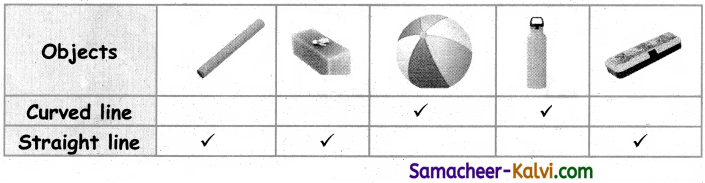Practice(Text Book Page No. 5)

Put a tick mark in the appropriate columns.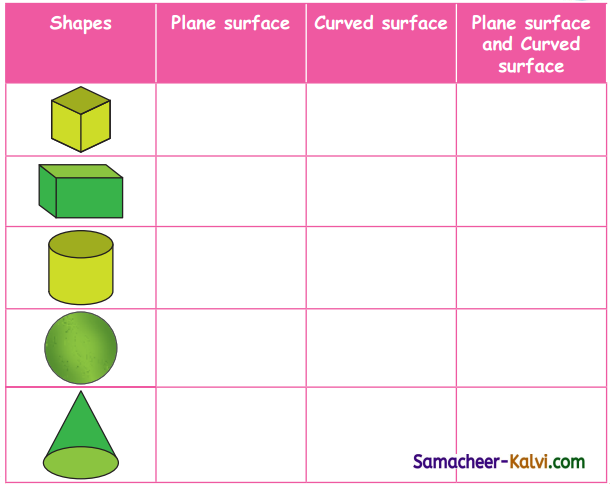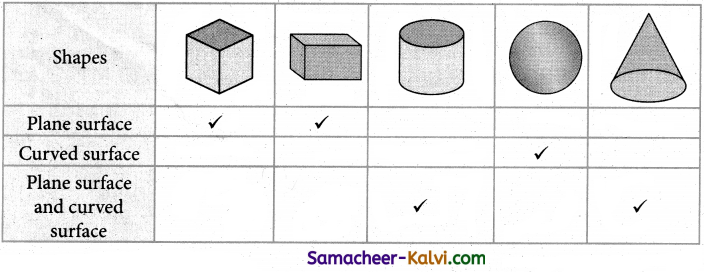Practice (Text Book Page No. 6)

Question 1.
Match the following.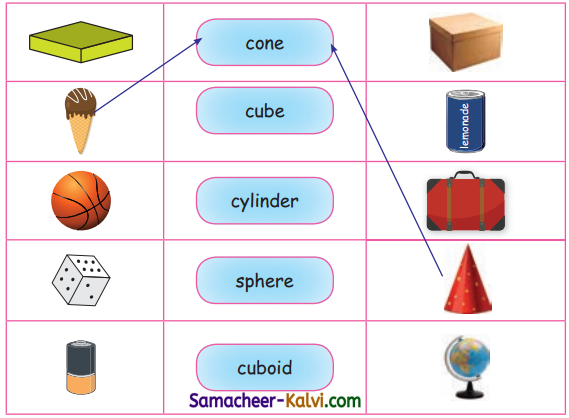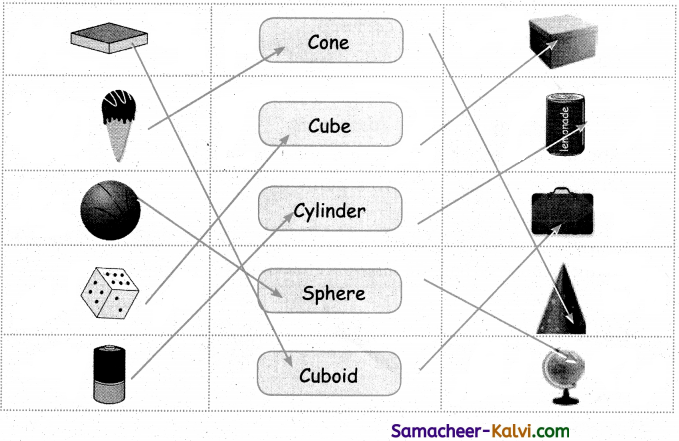Question 2.
Complete the following table by filling the properties of 2D and 3D shapes: